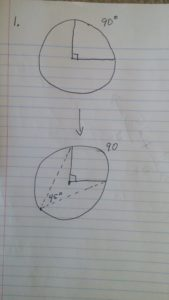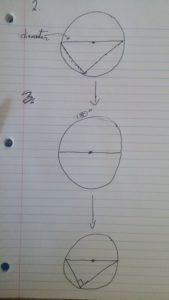# Inscribed angles

A common question in geometry class is finding inscribed angle. What is an inscribed angle? It’s an angle formed by two chords that have a vertex at one edge of a circle and distinct endpoints at somewhere else on the circle. This is in contrast to a central angle, which has a vertex at the center of the circle, from which two legs extend to the edges of the circle.In the picture above of problem 1, we see the top circle consists of a central angle that forms an arc of 90 degrees. Below I have added an inscribed angle that has endpoints matching those of the central angle. You can see that this angle is half of the angle of the central angle. This is an important rule to remember for geometry.In this second example (above), we start with a chord that spans the diameter and is a central angle because it goes through the center. It creates an arc of 180 degrees because it cuts the circle in half. At the bottom of the image I have added an inscribed angle of 90 degrees, demonstrating once again that they are half of central angles. You can think of this as the “flashlight effect.”